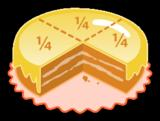Fraction (Mathematics)

# Fraction (mathematics)

to get instant updates about 'Fraction (Mathematics)' on your MyPage. Meet other similar minded people. Its Free!

X

Description:
Fractions (from , "broken") are numbers expressed as the ratio of two numbers, and are used primarily to express a comparison between parts and a whole.

The earliest fractions were reciprocals of integers: ancient symbols representing one part of two, one part of three, one part of four, and so on. A much later development were the common or "vulgar" fractions which are still used today (½, ⅝, ¾, etc.) and which consist of a numerator and a denominator, the numerator representing a number of equal parts and the denominator telling how many of those parts make up a whole.An example is 3/4, in which the numerator, 3, tells us that the fraction represents 3 equal parts, and the denominator, 4, tells us that 4 parts make up a whole.

A still later development was the decimal fraction, now called simply a decimal, in which the denominator is a power of ten, determined by the number of digit to the right of a decimal separator, the appearance of which (e.g., a period, a raised period (•), a comma) depends on the locale (for examples, see decimal separator). Thus for 0.75 the numerator is 75 and the denominator is 10 to the second power, viz. 100, because there are two digits to the right of the decimal separator.

A third kind of fraction still in common use is the percentage, in which the denominator is always 100. Thus 75% means 75/100.

Other uses for fractions are to represent ratios, and to represent division. Thus the...

No feeds found

AllPosting your question. Please wait!...